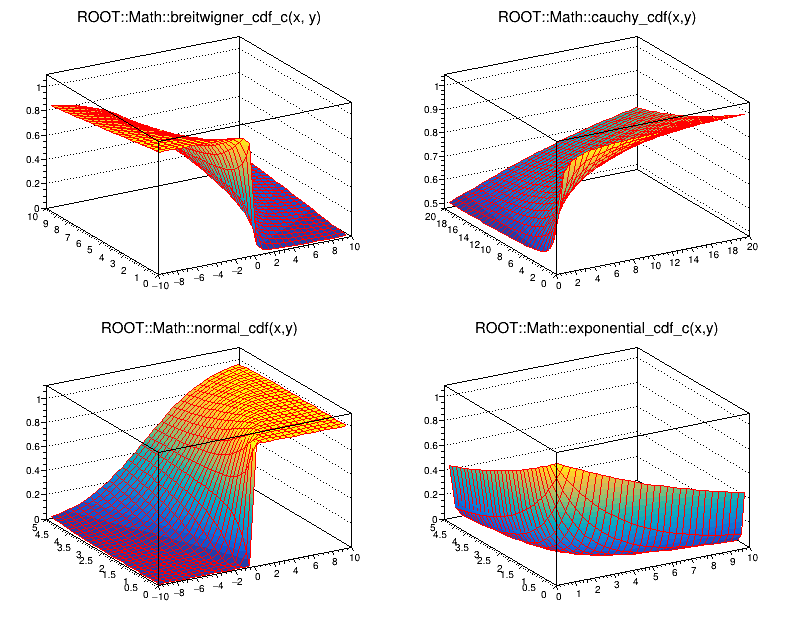# mathcoreCDF¶

Example describing how to use the different cumulative distribution functions in ROOT. The macro shows four of them with respect to their two variables. In order to run the macro type:

root  .x mathcoreCDF.C


Author: Lorenzo Moneta
This notebook tutorial was automatically generated with ROOTBOOK-izer from the macro found in the ROOT repository on Wednesday, August 17, 2022 at 09:34 AM.

In :
TF2 *f1a = new TF2("f1a","ROOT::Math::breitwigner_cdf_c(x, y)",-10,10,0,10);
TF2 *f2a = new TF2("f2a","ROOT::Math::cauchy_cdf(x,y)",0,20, 0,20);
TF2 *f3a = new TF2("f3a","ROOT::Math::normal_cdf(x,y)",-10,10,0,5);
TF2 *f4a = new TF2("f4a","ROOT::Math::exponential_cdf_c(x,y)",0,10,0,5);

TCanvas *c1 = new TCanvas("c1","c1",800,650);

c1->Divide(2,2);
c1->cd(1); f1a->SetLineWidth(1);
f1a->Draw("surf1");
c1->cd(2); f2a->SetLineWidth(1);
f2a->Draw("surf1");
c1->cd(3); f3a->SetLineWidth(1);
f3a->Draw("surf1");
c1->cd(4); f4a->SetLineWidth(1);
f4a->Draw("surf1");


Draw all canvases

In :
gROOT->GetListOfCanvases()->Draw()